# Chi-square test.pptx

29 de May de 2023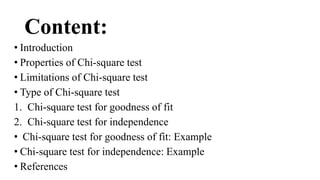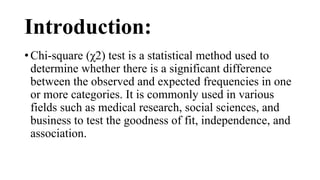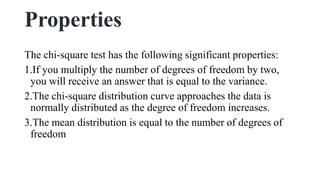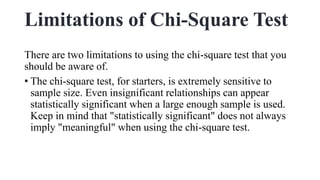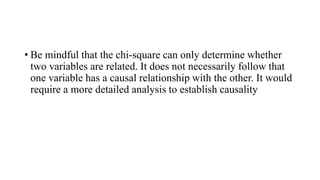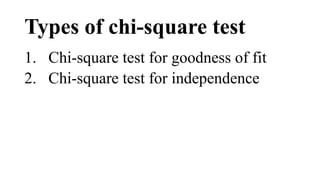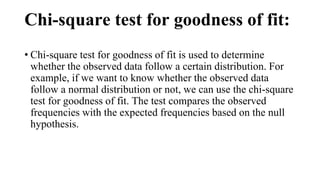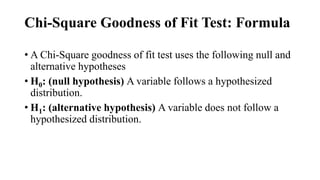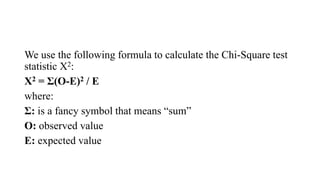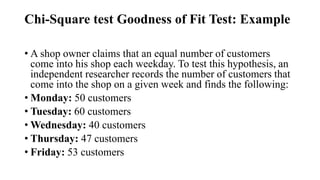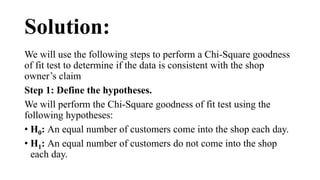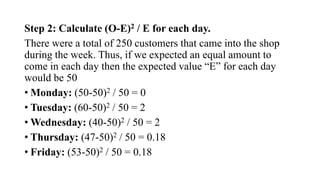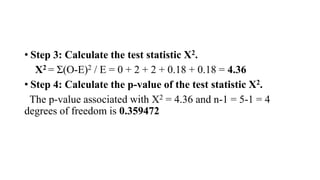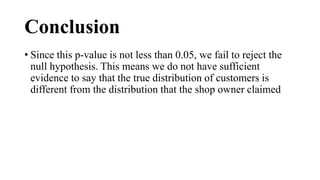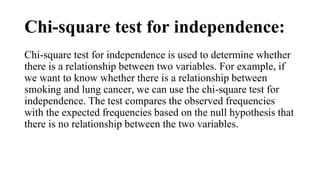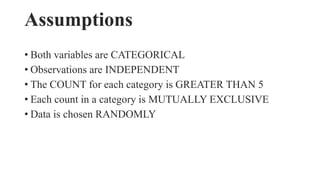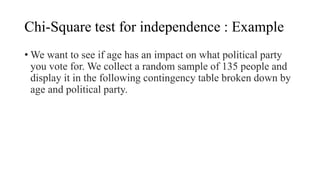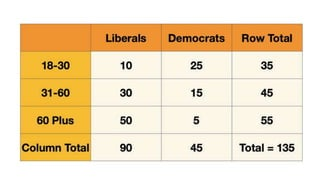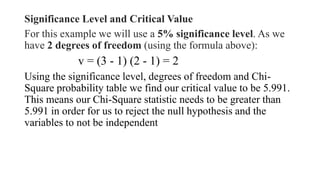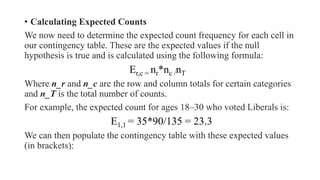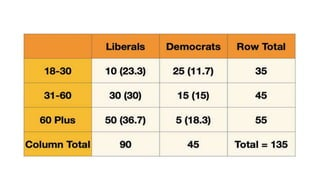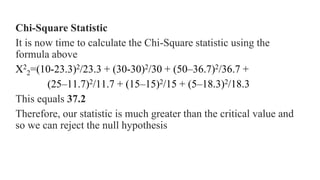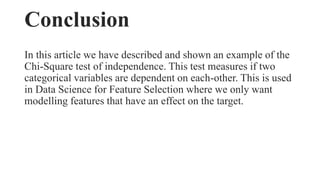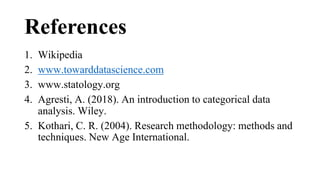1 de 26

### Chi-square test.pptx

• 1. ACHARYA NARENDRA DEVA UNIVERSITY OF AGRICULTURE & TECHNOLOGY, KUMARGANJ, AYODHYA (U.P.) 224229 Assignment on Chi-square test Course No : STAT-502 4(3+1) Course name : Statistical methods for applied sciences Presented to : Presented by : Dr. Vishal Mehta Vikas Yadav Assistant Professor Id. No. A-11153/19/22 Department of Agril. Statistics Ph. D. 1st Semester Soil Science and Agril. Chemistry
• 2. Content: • Introduction • Properties of Chi-square test • Limitations of Chi-square test • Type of Chi-square test 1. Chi-square test for goodness of fit 2. Chi-square test for independence • Chi-square test for goodness of fit: Example • Chi-square test for independence: Example • References
• 3. Introduction: • Chi-square (χ2) test is a statistical method used to determine whether there is a significant difference between the observed and expected frequencies in one or more categories. It is commonly used in various fields such as medical research, social sciences, and business to test the goodness of fit, independence, and association.
• 4. Properties The chi-square test has the following significant properties: 1.If you multiply the number of degrees of freedom by two, you will receive an answer that is equal to the variance. 2.The chi-square distribution curve approaches the data is normally distributed as the degree of freedom increases. 3.The mean distribution is equal to the number of degrees of freedom
• 5. Limitations of Chi-Square Test There are two limitations to using the chi-square test that you should be aware of. • The chi-square test, for starters, is extremely sensitive to sample size. Even insignificant relationships can appear statistically significant when a large enough sample is used. Keep in mind that "statistically significant" does not always imply "meaningful" when using the chi-square test.
• 6. • Be mindful that the chi-square can only determine whether two variables are related. It does not necessarily follow that one variable has a causal relationship with the other. It would require a more detailed analysis to establish causality
• 7. Types of chi-square test 1. Chi-square test for goodness of fit 2. Chi-square test for independence
• 8. Chi-square test for goodness of fit: • Chi-square test for goodness of fit is used to determine whether the observed data follow a certain distribution. For example, if we want to know whether the observed data follow a normal distribution or not, we can use the chi-square test for goodness of fit. The test compares the observed frequencies with the expected frequencies based on the null hypothesis.
• 9. Chi-Square Goodness of Fit Test: Formula • A Chi-Square goodness of fit test uses the following null and alternative hypotheses • H0: (null hypothesis) A variable follows a hypothesized distribution. • H1: (alternative hypothesis) A variable does not follow a hypothesized distribution.
• 10. We use the following formula to calculate the Chi-Square test statistic X2: X2 = Σ(O-E)2 / E where: Σ: is a fancy symbol that means “sum” O: observed value E: expected value
• 11. Chi-Square test Goodness of Fit Test: Example • A shop owner claims that an equal number of customers come into his shop each weekday. To test this hypothesis, an independent researcher records the number of customers that come into the shop on a given week and finds the following: • Monday: 50 customers • Tuesday: 60 customers • Wednesday: 40 customers • Thursday: 47 customers • Friday: 53 customers
• 12. Solution: We will use the following steps to perform a Chi-Square goodness of fit test to determine if the data is consistent with the shop owner’s claim Step 1: Define the hypotheses. We will perform the Chi-Square goodness of fit test using the following hypotheses: • H0: An equal number of customers come into the shop each day. • H1: An equal number of customers do not come into the shop each day.
• 13. Step 2: Calculate (O-E)2 / E for each day. There were a total of 250 customers that came into the shop during the week. Thus, if we expected an equal amount to come in each day then the expected value “E” for each day would be 50 • Monday: (50-50)2 / 50 = 0 • Tuesday: (60-50)2 / 50 = 2 • Wednesday: (40-50)2 / 50 = 2 • Thursday: (47-50)2 / 50 = 0.18 • Friday: (53-50)2 / 50 = 0.18
• 14. • Step 3: Calculate the test statistic X2. X2 = Σ(O-E)2 / E = 0 + 2 + 2 + 0.18 + 0.18 = 4.36 • Step 4: Calculate the p-value of the test statistic X2. The p-value associated with X2 = 4.36 and n-1 = 5-1 = 4 degrees of freedom is 0.359472
• 15. Conclusion • Since this p-value is not less than 0.05, we fail to reject the null hypothesis. This means we do not have sufficient evidence to say that the true distribution of customers is different from the distribution that the shop owner claimed
• 16. Chi-square test for independence: Chi-square test for independence is used to determine whether there is a relationship between two variables. For example, if we want to know whether there is a relationship between smoking and lung cancer, we can use the chi-square test for independence. The test compares the observed frequencies with the expected frequencies based on the null hypothesis that there is no relationship between the two variables.
• 17. Assumptions • Both variables are CATEGORICAL • Observations are INDEPENDENT • The COUNT for each category is GREATER THAN 5 • Each count in a category is MUTUALLY EXCLUSIVE • Data is chosen RANDOMLY
• 18. Chi-Square test for independence : Example • We want to see if age has an impact on what political party you vote for. We collect a random sample of 135 people and display it in the following contingency table broken down by age and political party.
• 20. Solution Hypothesis Lets start by stating our hypotheses: • H_0: Age has no impact on the political party you vote for. The two variables are independent. • H_1: Age does have an impact on the political party. The two variables are dependent.
• 21. Significance Level and Critical Value For this example we will use a 5% significance level. As we have 2 degrees of freedom (using the formula above): v = (3 - 1) (2 - 1) = 2 Using the significance level, degrees of freedom and Chi- Square probability table we find our critical value to be 5.991. This means our Chi-Square statistic needs to be greater than 5.991 in order for us to reject the null hypothesis and the variables to not be independent
• 22. • Calculating Expected Counts We now need to determine the expected count frequency for each cell in our contingency table. These are the expected values if the null hypothesis is true and is calculated using the following formula: Er,c = nr*nc /nT Where n_r and n_c are the row and column totals for certain categories and n_T is the total number of counts. For example, the expected count for ages 18–30 who voted Liberals is: E1,1 = 35*90/135 = 23.3 We can then populate the contingency table with these expected values (in brackets):
• 24. Chi-Square Statistic It is now time to calculate the Chi-Square statistic using the formula above Χ2 2=(10-23.3)2/23.3 + (30-30)2/30 + (50–36.7)2/36.7 + (25–11.7)2/11.7 + (15–15)2/15 + (5–18.3)2/18.3 This equals 37.2 Therefore, our statistic is much greater than the critical value and so we can reject the null hypothesis
• 25. Conclusion In this article we have described and shown an example of the Chi-Square test of independence. This test measures if two categorical variables are dependent on each-other. This is used in Data Science for Feature Selection where we only want modelling features that have an effect on the target.
• 26. References 1. Wikipedia 2. www.towarddatascience.com 3. www.statology.org 4. Agresti, A. (2018). An introduction to categorical data analysis. Wiley. 5. Kothari, C. R. (2004). Research methodology: methods and techniques. New Age International.